Скачать презентацию Design Of Water Distribution Network Of Asira Al-Shamalya

e56ac5a33874c71e38ee51f1f4dacd41.ppt

• Количество слайдов: 27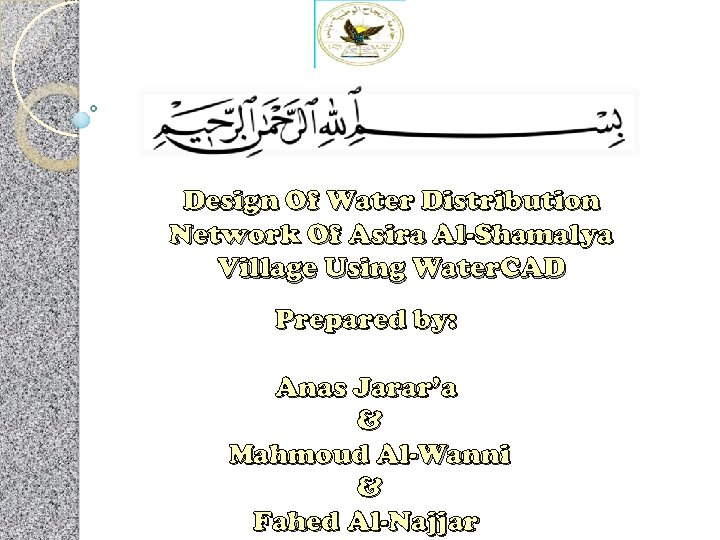Design Of Water Distribution Network Of Asira Al-Shamalya Village Using Water. CAD Prepared by: Anas Jarar’a & Mahmoud Al-Wanni & Fahed Al-Najjar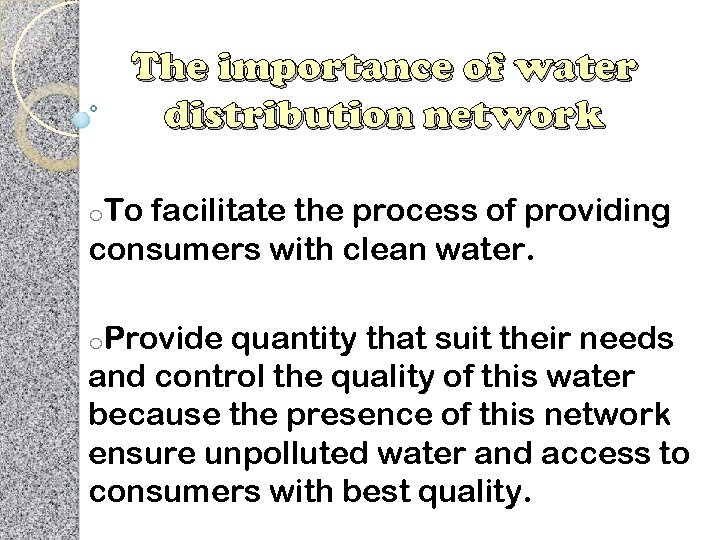The importance of water distribution network o. To facilitate the process of providing consumers with clean water. o. Provide quantity that suit their needs and control the quality of this water because the presence of this network ensure unpolluted water and access to consumers with best quality.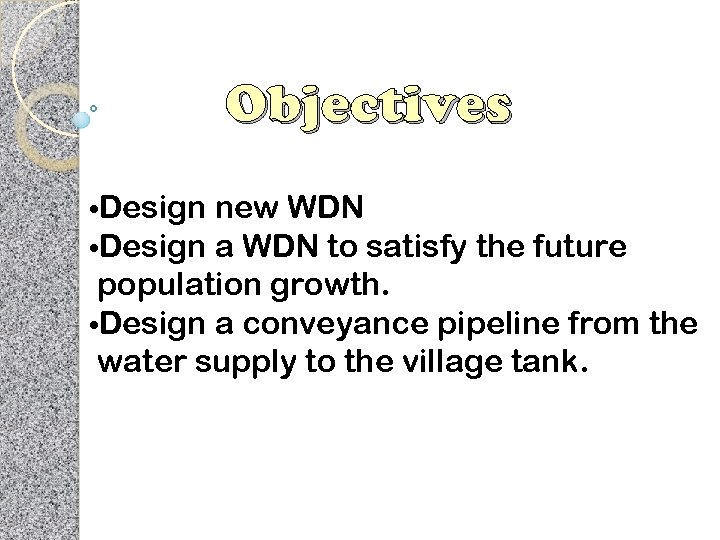Objectives • Design new WDN a WDN to satisfy the future population growth. • Design a conveyance pipeline from the water supply to the village tank.Methodology: .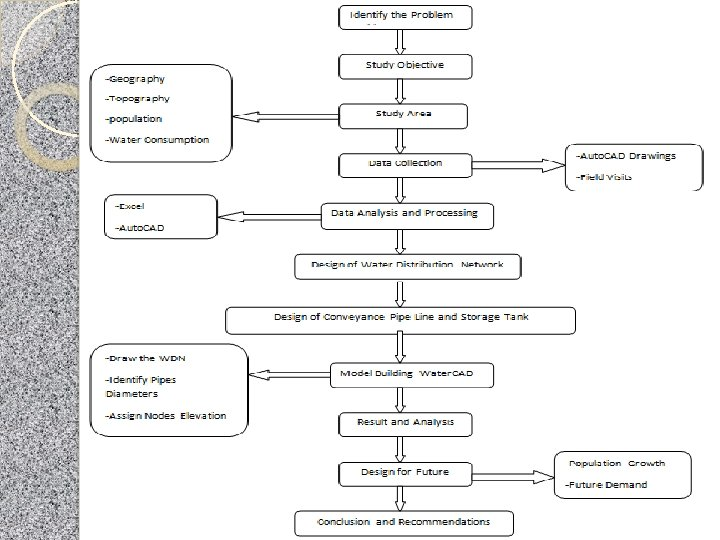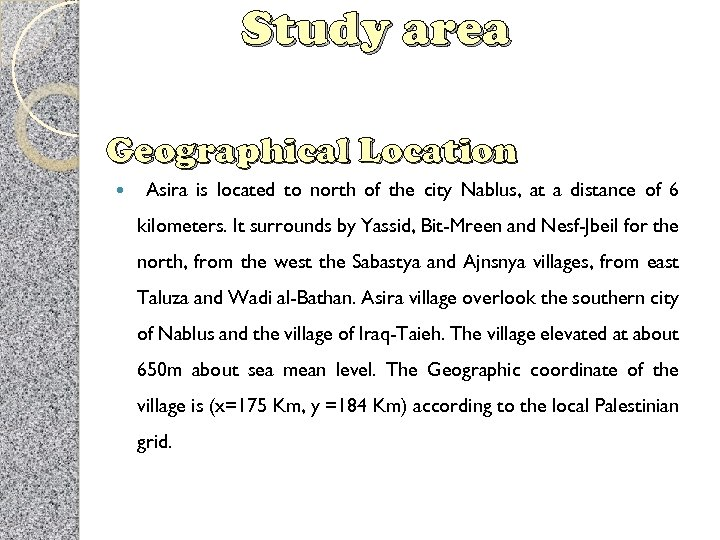Study area Geographical Location Asira is located to north of the city Nablus, at a distance of 6 kilometers. It surrounds by Yassid, Bit-Mreen and Nesf-Jbeil for the north, from the west the Sabastya and Ajnsnya villages, from east Taluza and Wadi al-Bathan. Asira village overlook the southern city of Nablus and the village of Iraq-Taieh. The village elevated at about 650 m about sea mean level. The Geographic coordinate of the village is (x=175 Km, y =184 Km) according to the local Palestinian grid.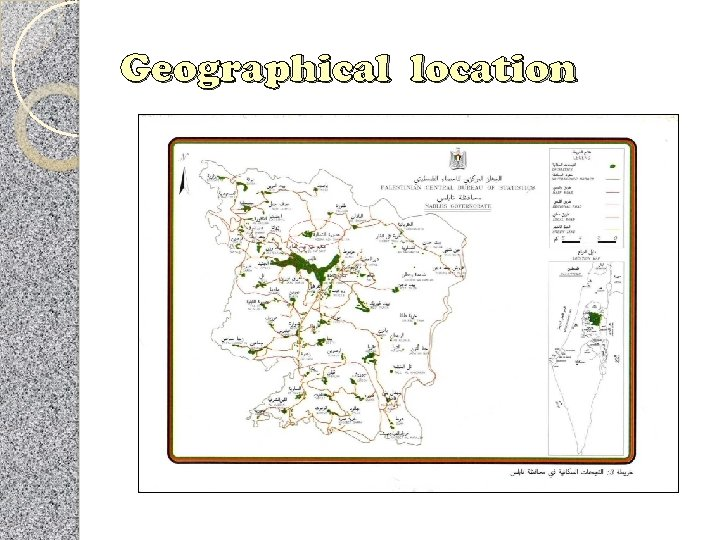Geographical location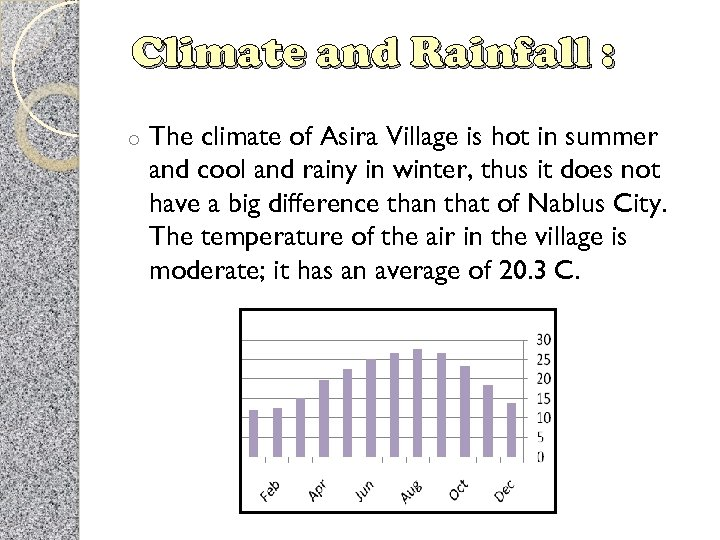Climate and Rainfall : o The climate of Asira Village is hot in summer and cool and rainy in winter, thus it does not have a big difference than that of Nablus City. The temperature of the air in the village is moderate; it has an average of 20. 3 C.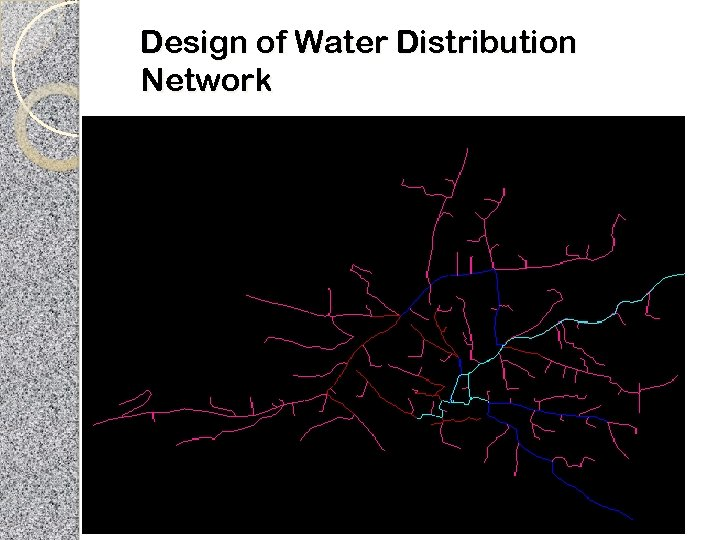Design of Water Distribution Network1 - Draw the Network by Water. CAD 2 - Calculations using Excel to determine the growth rate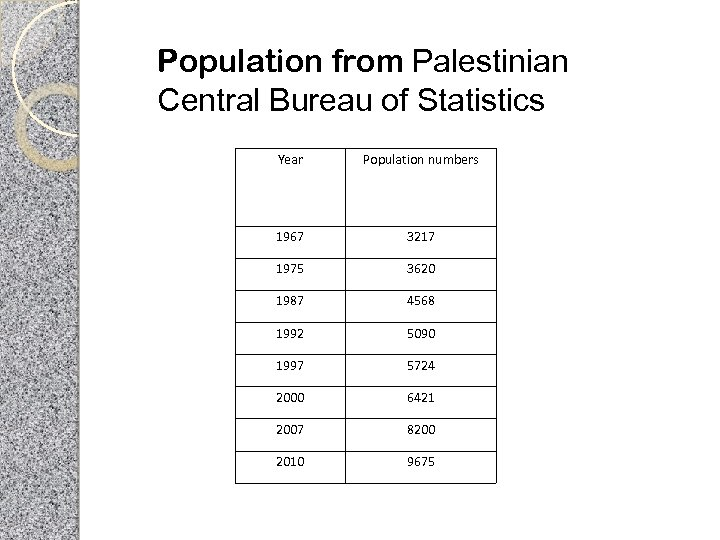Population from Palestinian Central Bureau of Statistics Year Population numbers 1967 3217 1975 3620 1987 4568 1992 5090 1997 5724 2000 6421 2007 8200 2010 9675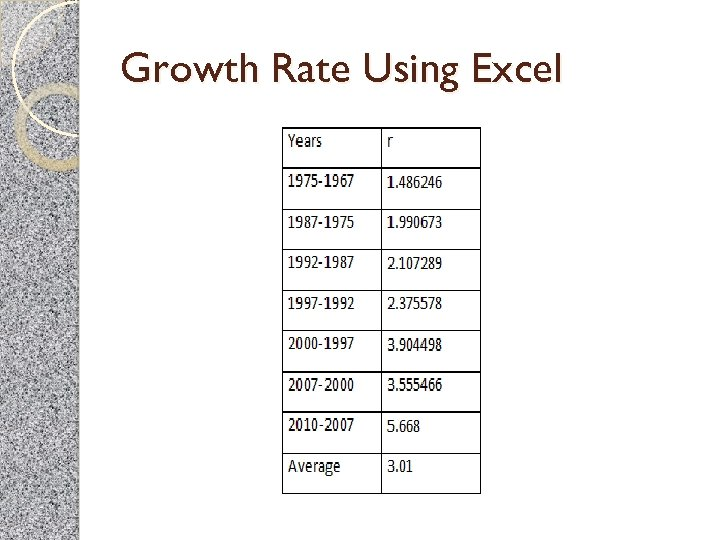Growth Rate Using Excel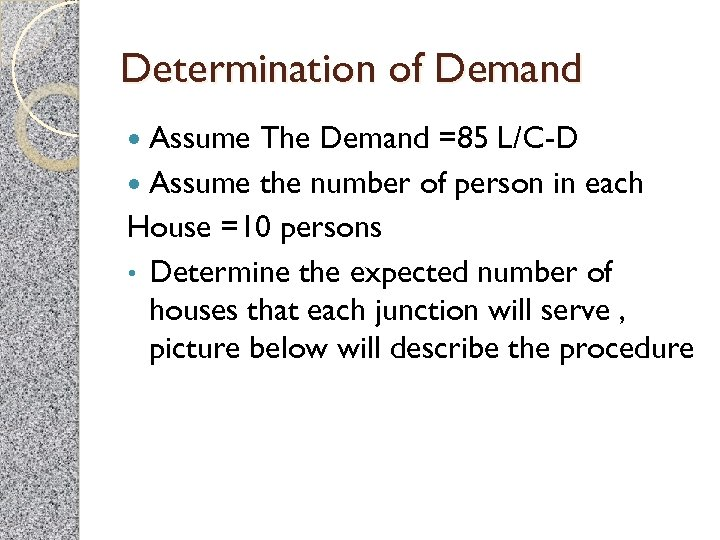Determination of Demand Assume The Demand =85 L/C-D Assume the number of person in each House =10 persons • Determine the expected number of houses that each junction will serve , picture below will describe the procedure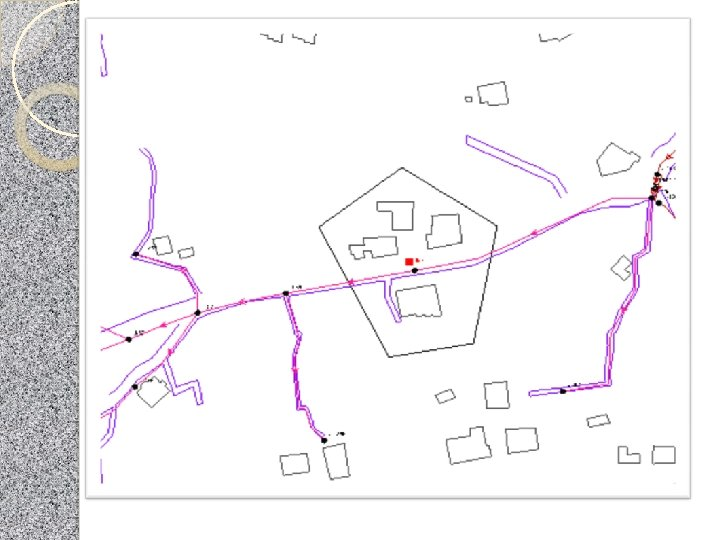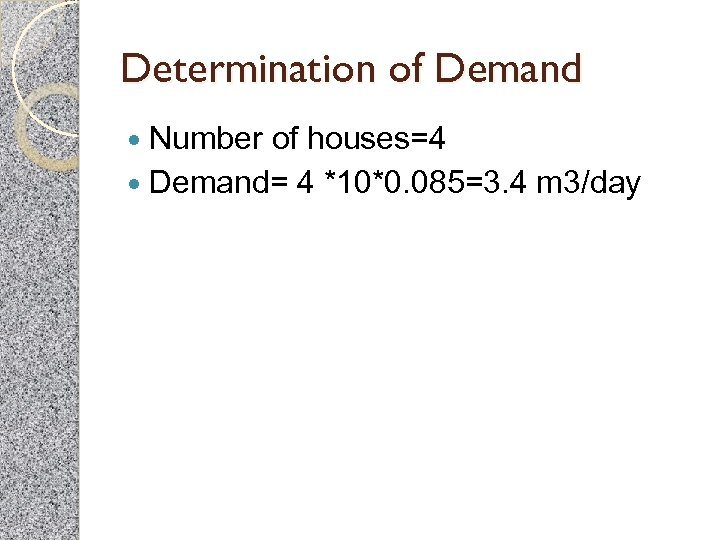Determination of Demand Number of houses=4 Demand= 4 *10*0. 085=3. 4 m 3/day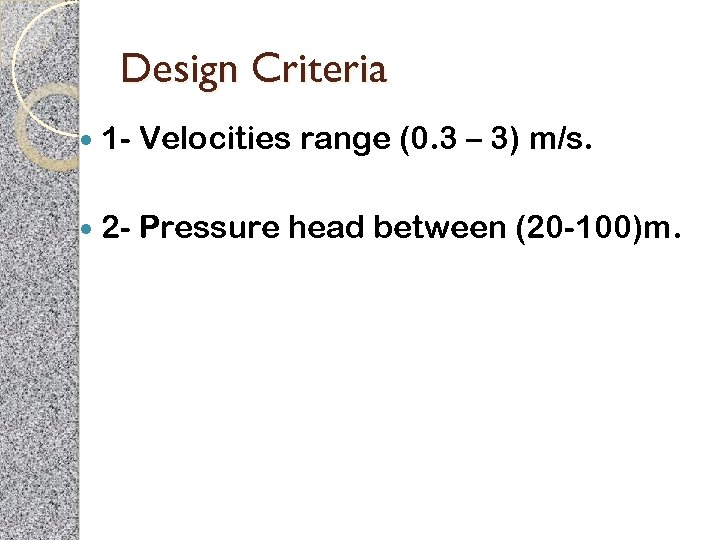Design Criteria 1 - Velocities range (0. 3 – 3) m/s. 2 - Pressure head between (20 -100)m.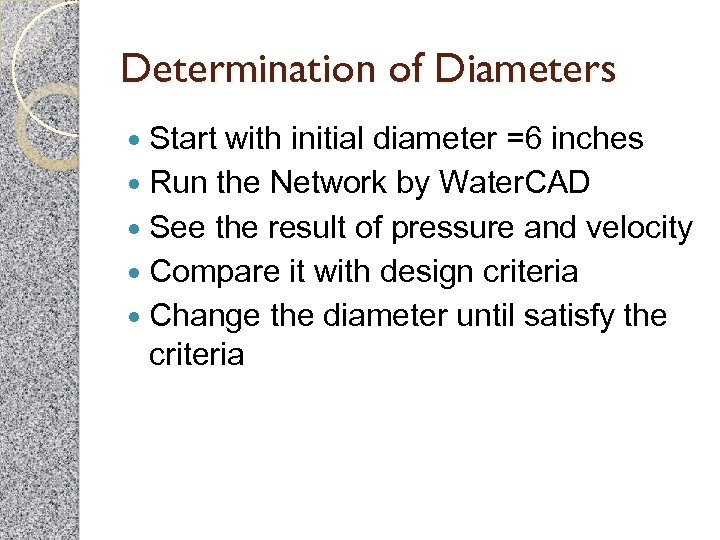Determination of Diameters Start with initial diameter =6 inches Run the Network by Water. CAD See the result of pressure and velocity Compare it with design criteria Change the diameter until satisfy the criteria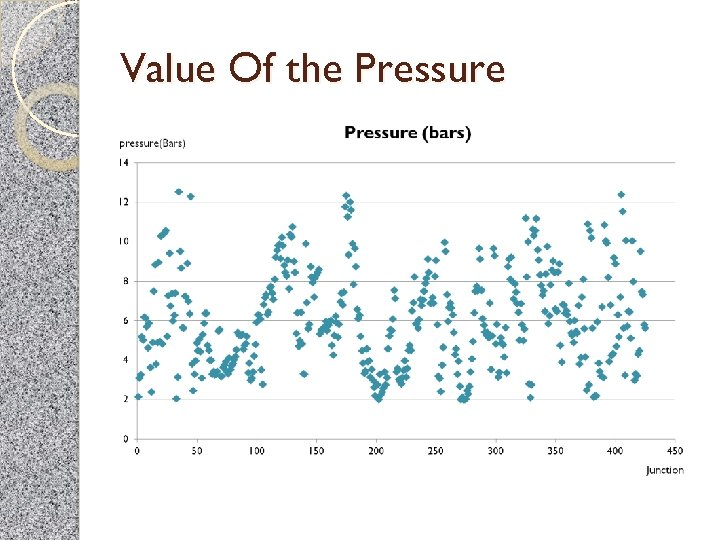Value Of the Pressure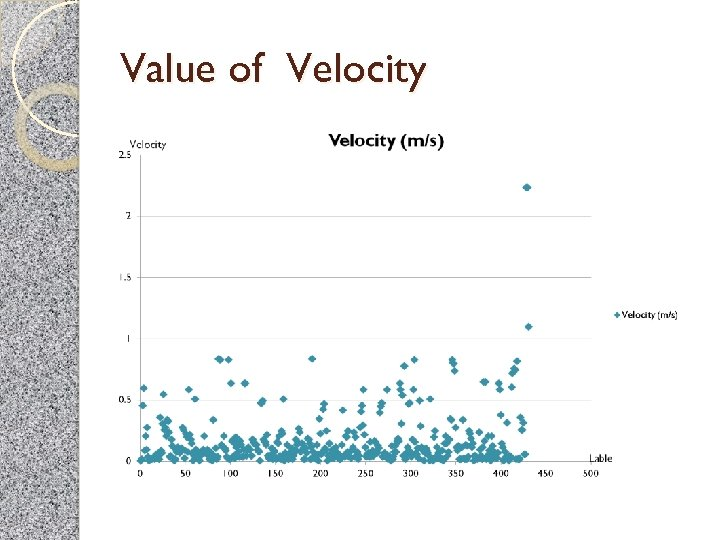Value of Velocity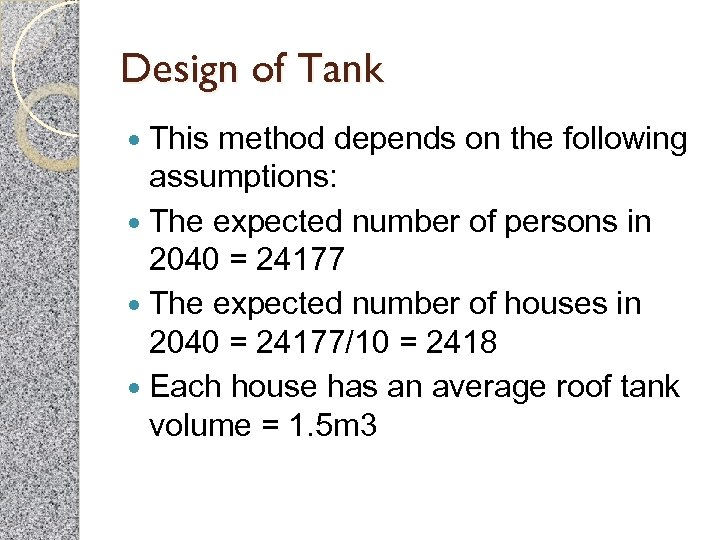Design of Tank This method depends on the following assumptions: The expected number of persons in 2040 = 24177 The expected number of houses in 2040 = 24177/10 = 2418 Each house has an average roof tank volume = 1. 5 m 3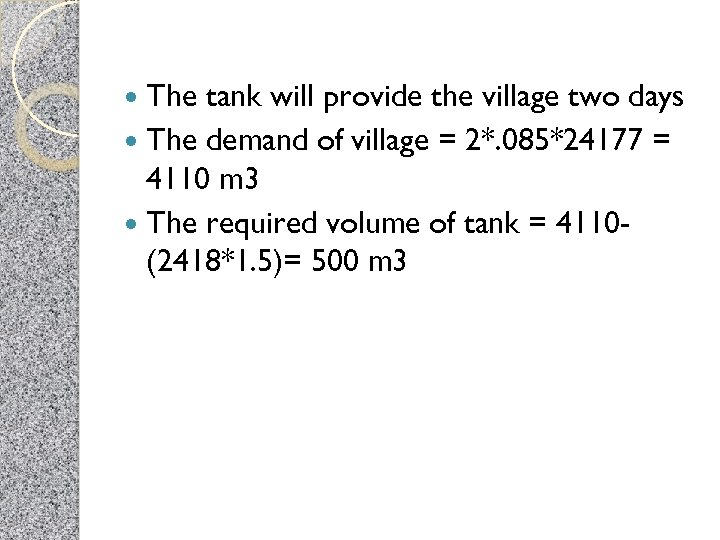The tank will provide the village two days The demand of village = 2*. 085*24177 = 4110 m 3 The required volume of tank = 4110(2418*1. 5)= 500 m 3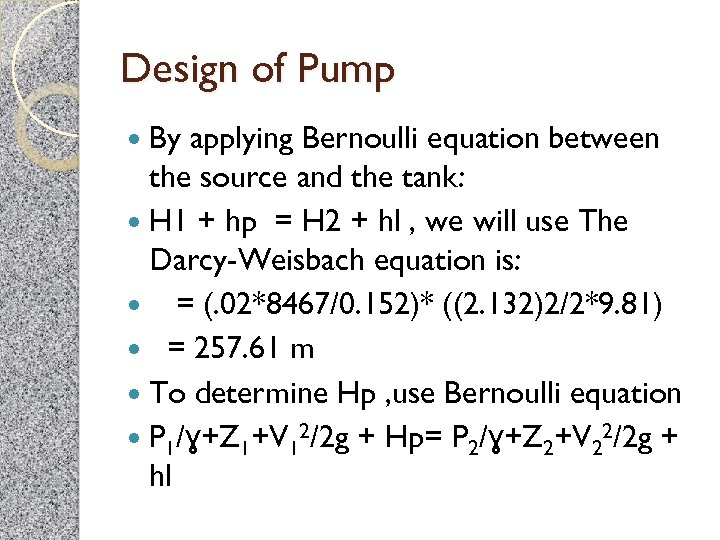Design of Pump By applying Bernoulli equation between the source and the tank: H 1 + hp = H 2 + hl , we will use The Darcy-Weisbach equation is: = (. 02*8467/0. 152)* ((2. 132)2/2*9. 81) = 257. 61 m To determine Hp , use Bernoulli equation P 1/ɣ+Z 1+V 12/2 g + Hp= P 2/ɣ+Z 2+V 22/2 g + hl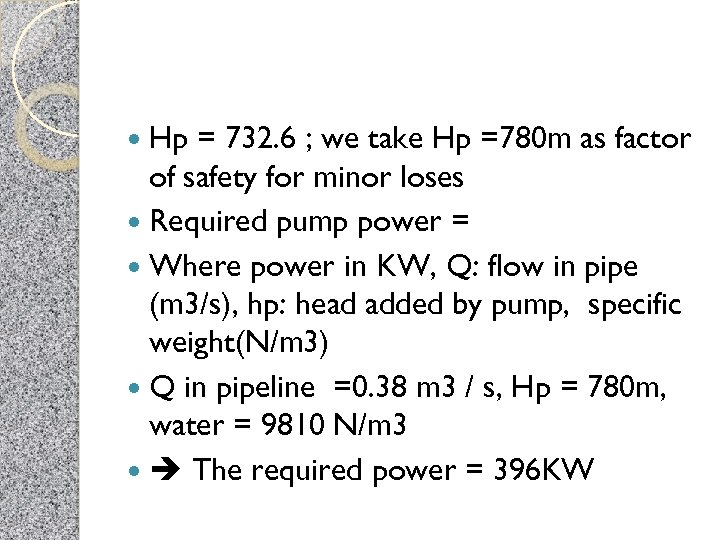Hp = 732. 6 ; we take Hp =780 m as factor of safety for minor loses Required pump power = Where power in KW, Q: flow in pipe (m 3/s), hp: head added by pump, specific weight(N/m 3) Q in pipeline =0. 38 m 3 / s, Hp = 780 m, water = 9810 N/m 3 The required power = 396 KW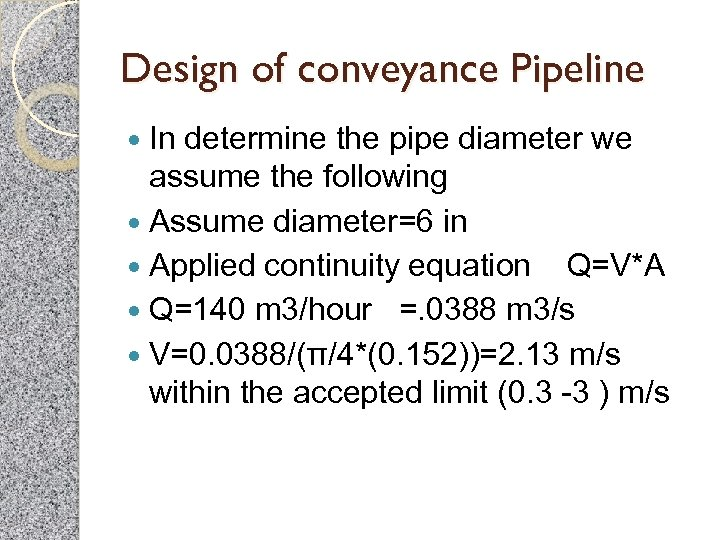Design of conveyance Pipeline In determine the pipe diameter we assume the following Assume diameter=6 in Applied continuity equation Q=V*A Q=140 m 3/hour =. 0388 m 3/s V=0. 0388/(π/4*(0. 152))=2. 13 m/s within the accepted limit (0. 3 -3 ) m/s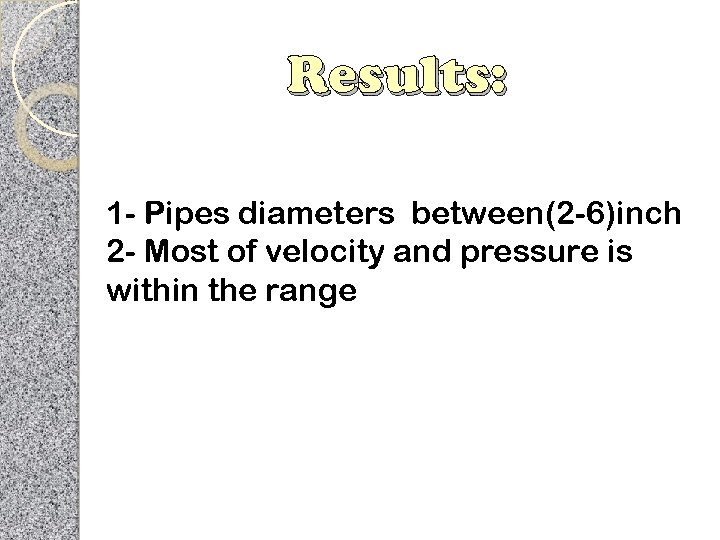Results: 1 - Pipes diameters between(2 -6)inch 2 - Most of velocity and pressure is within the range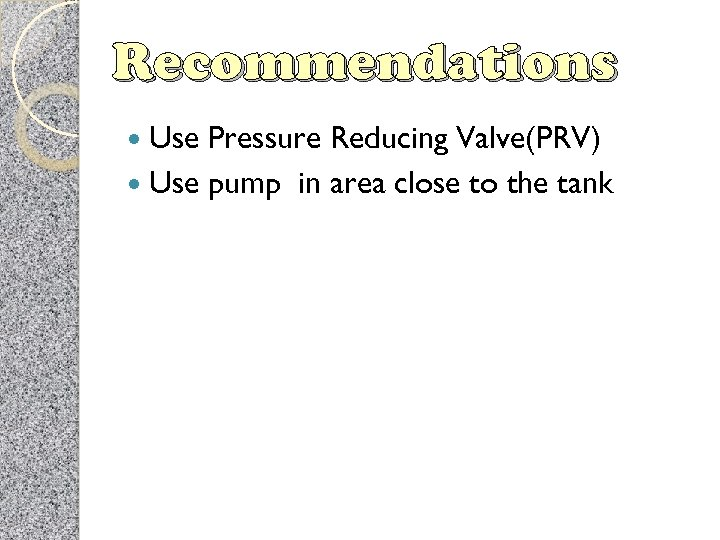Recommendations Use Pressure Reducing Valve(PRV) Use pump in area close to the tankTHANK YOU FOR LISTINENG